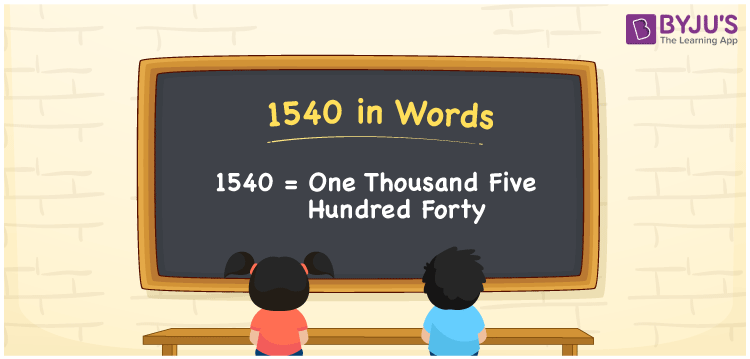# 1540 in words

1540 in words is written as One Thousand Five Hundred and Forty. 1540 represents the count or value. The article on Counting Numbers can give you an idea about count or counting. The number 1540 is used in expressions that relate to money, distance, length, year and others. Let us consider an example for 1540. “An overhead water tank has a capacity of One Thousand Five Hundred and Forty litres.” Another example is, “From the year One Thousand Five Hundred and Forty, the Sur Dynasty ruled Northern India.”

 1540 in words One Thousand Five Hundred and Forty One Thousand Five Hundred and Forty in Numbers 1540

## 1540 in English Words## How to Write 1540 in Words?

We can convert 1540 to words using a place value chart. The number 1540 has 4 digits, so let’s make a chart that shows the place value up to 4 digits.

 Thousands Hundreds Tens Ones 1 5 4 0

Thus, we can write the expanded form as:

1 × Thousands + 5 × Hundred + 4 × Ten + 0 × One

= 1 × 1000 + 5 × 100 + 4 × 10 + 0 × 1

= 1540

= One Thousand Five Hundred and Forty.

1540 is the natural number, succeeded by 1539 and preceded by 1541.

1540 in words – One Thousand Five Hundred and Forty.

Is 1540 an odd number? – No.

Is 1540 an even number? – Yes.

Is 1540 a perfect square number? – No.

Is 1540 a perfect cube number? – No.

Is 1540 a prime number? – No.

Is 1540 a composite number? – Yes.

## Solved Example

1. Write the number 1540 in expanded form

Solution: 1 × 1000 + 5 × 100 + 4 × 10 + 0 × 1

We can write 1540 = 1000 + 500 + 40 + 0

= 1 × 1000 + 5 × 100 + 4 × 10 + 0 × 1

## Frequently Asked Questions on 1540 in Words

Q1

### How to write 1540 in words?

1540 in words is written as One Thousand Five Hundred and Forty.
Q2

### State if True or False. 1540 is divisible by 2?

True. 1540 is divisible by 2.
Q3

### Is 1540 divisible by 10?

Yes. 1540 is divisible by 10.
Q4

### Is 1,540 an even number?

Yes, 1,540 is an even number.
Q5

### Is 1,540 a composite number?

Yes, 1,540 is a composite number.